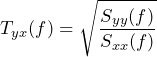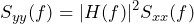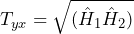# Transmissibility

June 2, 2021

A transfer function, H, can uncover a resonance or anti-resonance in a system. Transmissibility Tyx(f) of a signal pair x and y is as follows, where Syy is the PSD of y and Sxx is the PSD of x.

(1)One should consider using transmissibility if they are not concerned with the statistical relationship between x and y or if there is not a statistical relationship at all. It is also helpful for determining a value between Ĥ1 and Ĥ2, such as Ĥv.

### When not to Use Transmissibility

Transmissibility is not the best option if the goal is complex-valued system response data. Unlike all other transfer functions, transmissibility is real-valued and not complex-valued.

• Tyx(f) ≜ Ŝyy(f) / Ŝxx(f) (real ÷ real = real)
• Ĥ1(f) ≜ Ŝyy(f) / Ŝyx(f) (real ÷ complex = complex)

One should also not use transmissibility if they are concerned with the statistical relationship between x and y. Unlike all other transfer functions, transmissibility does not directly consider cross-statistical properties between x and y, it only uses the auto-statistical properties of x with x and y with y.

In particular, Tyx does not use the cross-spectral density of x and y; rather, it only uses the auto-spectral density (PSD) of x and PSD of y.

### Insights

1. For a linear time invariant (LTI) system with frequency response H(f):

(2)In the case of an LTI system, the transmissibility of x and y is equivalent to the magnitude of the frequency response |H(f)|.

1. Tyx is the geometric mean of Ĥ1 and Ĥ2 because Tyx is, in some sense, between Ĥ1 and Ĥ2.

(3)If there is noise on both input and output, then Tyx is an alternative to Ĥv, which is also between Ĥ1 and Ĥ2.